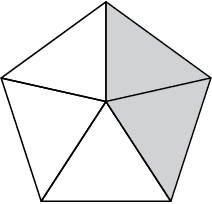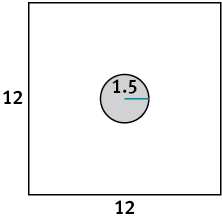1. Math
2. Geometry
3. 1 liam made a 3 3 target on a...

# Question: 1 liam made a 3 3 target on a...

###### Question details

1. Liam made a 3 × 3 target on a 10 × 10 dartboard.

What is the probability that a dart will hit Liam's target?

 93% 9% 3% 30%

2. Darts are thrown at random at each dartboard. Find the probability that the dart will land in the shaded region. Write your answer as a percent.________________________

3. Darts are thrown at random at each dartboard. Find the probability that the dart will land in the shaded region. Write your answer as a decimal.4. Darts are thrown at random at each dartboard. Find the probability that the dart will land in the shaded region. Write your answer as a decimal.5.. Olivia made a 6 × 6 target on a 10 × 10 dartboard. She said that the probability of a dart landing in her target area would be twice as great as the probability that a dart will land in Liam's target area. Do you agree?

 Yes; the dartboard is identical to Liam's but scaled up by a factor of two No; Olivia’s target area will be 4 times Liam’s target area, so the hit probability will be 4 times as great Yes; the area of the target is twice as big, so the probability of a hit is twice as much No; Olivia's reasoning is not taking into account the diminished non-target area

.

6. A spinner (shown) with the numbers from 1 to 12 is spun once. What is the probability that the result is an even number?75% 25% 1/2 3/12

7. What is the probability that the result is a prime number?

 1/12 5/12 50% 66 2/3%

8. What is the probability that the number is less than 13? _____________

9. What is the probability that the number is a multiple of 5?

 5/12 1/6 0 1/12

10. The members of the Math Club are designing dartboards. They experimented with different shapes. For which dartboard is the probability of hitting the bull's-eye the least? Note that the dartboards are not drawn to scale.###### Solution by an expert tutor